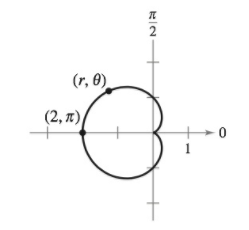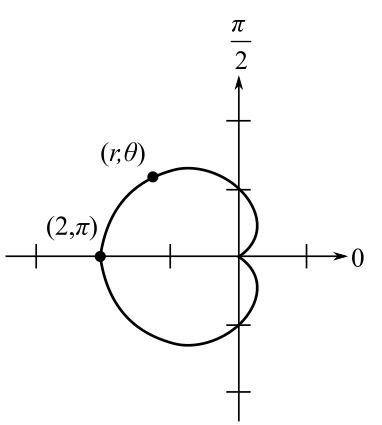Chapter 12, Problem 6PS

Chapter
Section
Textbook Problem
1 views

# CardioidsConsider the cardioids r = 1 − cos θ ,       0 ≤ θ ≤ 2 π as shown in the figure. Let s ( θ ) be the arc length from the point ( 2 , π ) on the cardioids to the point ( r , θ ) , and let p ( θ ) = 1 / K be the radius of curvature at the point ( r , θ ) . Show that s and p are related by the equation s 2 + 9 p 2 = 16 . (This equation is called a natural equation for the curve.)To determine

To prove: The s and ρ are related by the equation s2+9ρ2=16 where, the cardioids equation is, r(θ)=1cosθ for 0θ2π. s(θ) be the arc length from the point (2,π) on cardioids point (r,θ). The radius of curvature ρ(θ)=1K at the point (r,θ) as shown in the figure given below:Explanation

Given: Thecardioids equation is, r(θ)=1cosθ for 0θ2π. s(θ) be the arc length from the point (2,π) on cardioids point (r,θ). The radius of curvature ρ(θ)=1K at the point (r,θ). The figure is given below,

Proof: The one arch of the cycloid is,

r(θ)=1cosθ

As, x=rcosθ and y=rsinθ. Find these coordinate,

x=(1cosθ)cosθ=cosθcos2θ

And

y=(1cosθ)sinθ=sinθcosθsinθ=sinθ12sin2θ

Here, x(θ)=cosθcos2θ and y(θ)=sinθ12sin2θ.

Considering the function, x(θ),

x(θ)=cosθcos2θ

Differentiating the above given function,

x(θ)=sinθ2cosθ(sinθ)=sinθ+sin2θ …… (1)

Considering the function y(θ),

y(θ)=sinθ12sin2θ

Differentiating the above function,

y(θ)=cosθ12cos2θ(2)=cosθcos2θ …… (2)

The magnitude of position vector,

r=[x(θ)]2+[y(θ)]2=(sinθ+sin2θ)2+(cosθcos2θ)2=sin2θ2sinθsin2θ+sin22θ+cos2θ2cos2θcosθ+cos22θ=1+12(sinθsin2θ+cos2θcosθ)

Simplifying the above equation,

r=22cos(2θθ)=2(1cosθ)=2(2sin2θ2)=2sinθ2

From the given condition, the arc length between the point (2,π) to (r,θ). So, the arc length is defined for π to θ

### Still sussing out bartleby?

Check out a sample textbook solution.

See a sample solution

#### The Solution to Your Study Problems

Bartleby provides explanations to thousands of textbook problems written by our experts, many with advanced degrees!

Get Started